# Parametric Plots

A parametric equation defines a group of quantities as functions of one or more independent variables called parameters. Parametric equations are commonly used to express the coordinates of the points that make up a geometric object such as a curve or surface, in which case the equations are collectively called a parametric representation or parameterization (British English: "parametrisation") of the object. Mathematica has a dedicated command for these purposes: ParametricPlot.

You can display many functions using implicit methods. Explicitly defined functions can be plotted using the regular Plot command.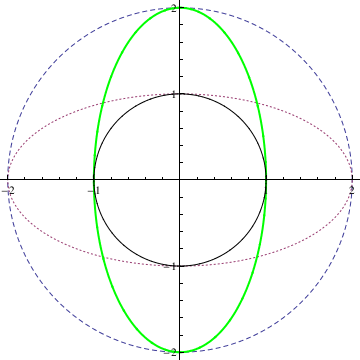Circles and ellipses. Labeled[ParametricPlot[{{2 Cos[t], 2 Sin[t]}, {2 Cos[t], Sin[t]}, {Cos[t], 2 Sin[t]}, {Cos[t], Sin[t]}}, {t, 0, 2 Pi}, PlotStyle -> {Dashed, Dashing[Tiny], Directive[Thick, Green], Black}], "Circles and elipses"] You can use the option Plotlegent to identify equations in use. ParametricPlot[{{2 Cos[t], 2 Sin[t]}, {2 Cos[t], Sin[t]}, {Cos[t], 2 Sin[t]}, {Cos[t], Sin[t]}}, {t, 0, 2 Pi}, PlotStyle -> {Dashed, Dashing[Tiny], Directive[Thick, Green], Black}, PlotLegends -> "Expressions"]

You can enjoy using a newer Mathematica package: NotebookEmbedder from Wolfram Cloud notebooks on websites. An HTML Mathematica package, NotebookEmbedder, permits you to place notebooks stored in the cloud in your website. Wolfram offers a wide range of options.

# The input element

Click the "Submit" button and the form-data will be sent to Wolfram Cloud at this specific URL to generate a plot and return the result as a PNG image".

# Alternate view in animation form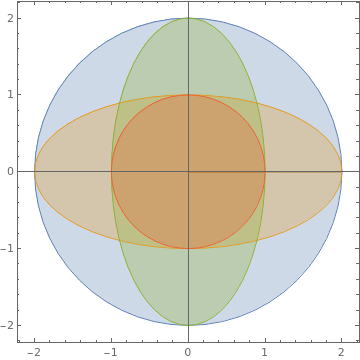Circles and ellipses with overlapping. Or you can make a similar plot with overlapping regions: ParametricPlot[{{2 r Cos[t], 2 r Sin[t]}, {2 r Cos[t], r Sin[t]}, {r Cos[t], 2 r Sin[t]}, {r Cos[t], r Sin[t]}}, {t, 0, 2 Pi}, {r, 0, 1}, Mesh -> False]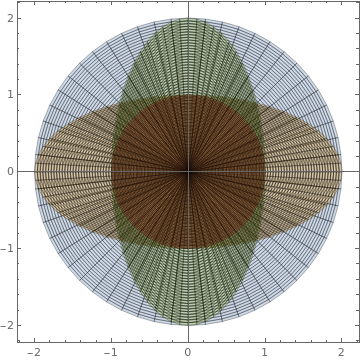Circles plotted with Mesh. Another option: ParametricPlot[{{2 r Cos[t], 2 r Sin[t]}, {2 r Cos[t], r Sin[t]}, {r Cos[t], 2 r Sin[t]}, {r Cos[t], r Sin[t]}}, {t, 0, 2 Pi}, {r, 0, 1}, Mesh -> All]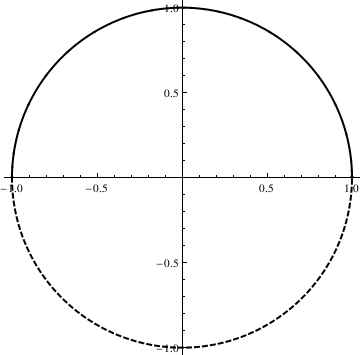Circles with distinct curve styles. ParametricPlot[{Cos[t], Sin[t]}, {t, 0, 2 Pi}, PlotStyle -> Directive[Thick, Black], Mesh -> {Range[0, 2 Pi, Pi]}, MeshShading -> {Dashed, {}}]

We can place our plots in a row:

pp1 = Labeled[ ParametricPlot[{{2 r Cos[t], 2 r Sin[t]}, {2 r Cos[t], r Sin[t]}, {r Cos[t], 2 r Sin[t]}, {r Cos[t], r Sin[t]}}, {t, 0, 2 Pi}, {r, 0, 1}, Mesh -> False], "Overlapping regions"];
pp2 = Labeled[ ParametricPlot[{{2 r Cos[t], 2 r Sin[t]}, {2 r Cos[t], r Sin[t]}, {r Cos[t], 2 r Sin[t]}, {r Cos[t], r Sin[t]}}, {t, 0, 2 Pi}, {r, 0, 1}, Mesh -> All], "Showing mesh elements used in sampling"];
pp3 = Labeled[ ParametricPlot[{Cos[t], Sin[t]}, {t, 0, 2 Pi}, PlotStyle -> Directive[Thick, Black], Mesh -> {Range[0, 2 Pi, Pi]}, MeshShading -> {Dashed, {}}], "Segments of curves with different styles"];
GraphicsRow[{pp1, pp2, pp3}, ImageSize -> 700]

Sin waves, parabolas, circles, arcs and ellipses are just special cases of the more general concept of "curve." Here are a circle and an ellipse. Note that the independent variable, t, must be the same within each equation. Both x and y must be parameterized by t, one may not, for instance, parameterize x by t and y by s.

x := Cos[t]; y := Sin[t];
pp4 = ParametricPlot[{x, y}, {t, 0, 2 Pi}];
pp5 = ParametricPlot[{2*x, y}, {t, 0, 2 Pi}];
GraphicsRow[{pp4, pp5}]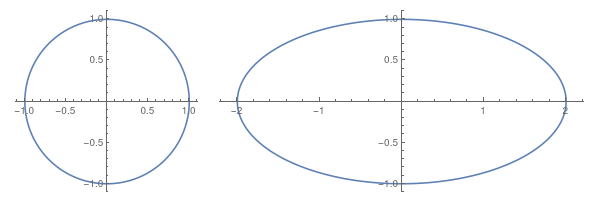Also, we can plot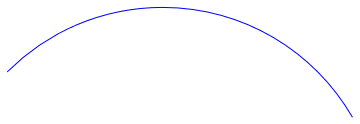Part of the ellipse. Actually, there is a special command in Mathematica that can be used to plot circles and ellipses: Circle[{x,y},r] gives a circle of radius r centered at {x,y}. Circle[{x,y}] represents a circle of radius 1. Circle[{x,y},{r_x , r_y}] gives an axis-aligned ellipse with semi-axes length r_x and r_y. Circle[{x,y},..., {theta_1 , theta_2}] gives a a circular or ellipse arc from angle theta_1 to theta_2 Graphics[{Blue, Circle[{0, 1}, 2, {Pi/6, 3*Pi/4}]}]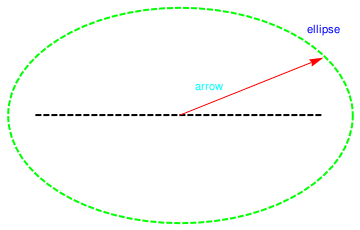Ellipse/circle with text. R = Graphics[{Green, Thick, Dashed, Circle[{1, 2}, {1.2, 0.75}], {Blue, Inset["ellipse", {2, 2.6}]}, {Black, Line[{{0, 2}, {2, 2}}]}}] a = Graphics[{Red, Arrow[{{1, 2}, {2, 2.4}}], {Cyan, Text[arrow, {1.2, 2.2}]}}] Show[R, a]

There are special commands in Mathematica that can be used to plot circles and ellipses:

Circle[{x, y}, r] gives a circle of radius r centered at {x, y} .
Circle[{x, y}] represents a circle of radius 1.
Circle[{x, y}, {r_x, r_y}] gives an axis - aligned ellipse with semi - axes length r_x and r_y .
Circle[{x, y}, ..., {theta_ 1, theta_ 2}] gives a a circular or ellipse arc from angle theta_ 1 to theta_ 2

Labeled[Graphics[{Blue, Thick, Circle[{0, 1}, 2, {Pi/6, 3*Pi/4}]}], "Part of an ellipse"]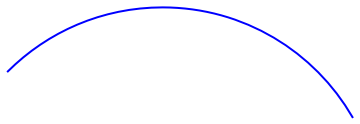pp6 = Graphics[{Green, Thick, Dashed, Circle[{1, 2}, {1.2, 0.75}], {Blue, Inset["ellipse", {2, 2.6}]}, {Black, Line[{{0, 2}, {2, 2}}]}}];
a = Graphics[{Red, Arrow[{{1, 2}, {2, 2.4}}], {Cyan, Text["arrow", {1.2, 2.2}]}}];
Labeled[Show[pp6, a], "Ellipse with text"]

To visualize spherical coordinates, use the following codes: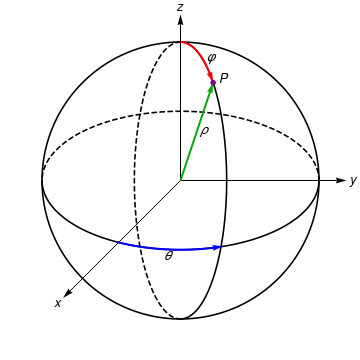Sphere. circle[x_] = {Cos[x], Sin[x]}; ellipsePhi[x_, a_: - Pi/2] = {Cos[x - a]/3, Sin[x + a]}; ellipseTheta[x_, a_: 0] = {Cos[x + a], Sin[-x - a]/2}; ParametricPlot[circle[x], {x, 0, 2 Pi}, PlotStyle -> Black, Epilog -> First /@ {(*Ellipses*) ParametricPlot[{ellipsePhi[x], ellipsePhi[-x], ellipseTheta[-x], ellipseTheta[x]}, {x, 0, Pi}, PlotStyle -> {{Black, Dashed}, Black}],(*Co-ordinate axes*) Graphics[ Table[GeometricTransformation[{Arrowheads[0.03], Arrow[{{0, 0}, {1.2, 0}}]}, ReflectionMatrix[circle[x]]], {x, {Pi/2, -Pi/4, Pi/8}}]], (*mark point,rho,phi& theta directions*) ParametricPlot[{ellipsePhi[x, Pi/2], ellipseTheta[-x, 13 Pi/20]}, {x, 0, Pi/4}, PlotStyle -> {{Red, Thick}, {Blue, Thick}}] /. Line[x__] :> Sequence[Arrowheads[0.03], Arrow[x]], Graphics[{{Directive[Darker@Green, Thick], Arrowheads[0.03], Arrow[{{0, 0}, ellipsePhi[-3 Pi/4]}]}, {Directive[Purple], Disk[ellipsePhi[-3 Pi/4], 0.02]}}], (*text*) Graphics[{Text[Style["x", Italic, Larger], 1.25 circle[5 Pi/4]], Text[Style["y", Italic, Larger], 1.25 circle], Text[Style["z", Italic, Larger], 1.25 circle[Pi/2]], Text[Style["\[Rho]", Italic, Larger], 0.4 circle[4 Pi/11]], Text[Style["\[CurlyPhi]", Italic, Larger], 1.1 ellipsePhi[Pi + Pi/5]], Text[Style["\[Theta]", Italic, Larger], 1.1 ellipseTheta[13 Pi/20 - Pi/8]], Text[Style["P", Italic, Larger], 1.2 ellipsePhi[-3 Pi/4 + Pi/24]]}] }, Axes -> False, PlotRange -> 1.3 {{-1, 1}, {-1, 1}} ]

This alternative solution has the advantage of being created using 3D directives. As such, it was easy to wrap inside a Manipulate and you can drag it with your mouse to change the viewpoint:
Manipulate[ Module[{x = Sin[\[Phi]] Cos[\[Theta]], y = Sin[\[Phi]] Sin[\[Theta]], z = Cos[\[Phi]]}, Show[ParametricPlot3D[{{Cos[t], Sin[t], 0}, {0, Sin[t], Cos[t]}, {Sin[t], 0, Cos[t]}}, {t, 0, 2 \[Pi]}, PlotStyle -> Black, Boxed -> False, Axes -> False, AxesLabel -> {"x", "y", "z"}], ParametricPlot3D[0.5*{Cos[t], Sin[t], 0}, {t, 0, \[Theta]}], ParametricPlot3D[ RotationTransform[\[Theta], {0, 0, 1}][{Sin[t]/2, 0, Cos[t]/2}], {t, 0, \[Phi]}], Graphics3D[{{{Blue, Thick, Arrow[{{0, 0, 0}, #}] & /@ {{1, 0, 0}, {0, 1, 0}, {0, 0, 1}, {x, y, z}}}, {Opacity[0.1], Red, Polygon[{{0, 0, 0}, {x, y, 0}, {x, y, z}}], Green, Polygon[{{0, 0, 0}, {x, 0, 0}, {x, y, 0}}]}}, {Opacity[0.05], Sphere[{0, 0, 0}]}, {Text["O", {-.03, -.03, -.03}], Text["X", {1.1, 0, 0}], Text["Q", {x, y, 0}, {1, 1}], Text["P", {x, y, z}, {0, -1}], Text["Y", {0, 1.1, 0}], Text["Z", {0, 0, 1.1}], Text["r", {x/2, y/2, 0}, {1, 1}], Text["\[Theta]", {Cos[\[Theta]/2]/2, Sin[\[Theta]/2]/2, 0}, {1, 1}], Text["\[Phi]", RotationTransform[\[Theta], {0, 0, 1}][{Sin[\[Phi]/2]/2, 0, Cos[\[Phi]/2]/2}], {1, 1}]}}]]], {{\[Phi], \[Pi]/4}, 0.01, \[Pi]/2}, {{\[Theta], \[Pi]/4}, 0.01, 2 \[Pi]}]

Another way to think about parametric plots is that it allows one to "sneak" a fourth variable into a plot of a three dimensional figure. To visualize this using spherical coordinates, below you see three axes, x, y and z, each parameterized by a fourth variable, t:

Alternative plot:

circle[t_] = {Cos[t], Sin[t]};
ellipsePhi[t_, a_ : -Pi/2] = {Cos[t - a]/3, Sin[t + a]};
ellipseTheta[t_, a_ : 0] = {Cos[t + a], Sin[-t - a]/2};
pp7 = ParametricPlot[{circle[t], ellipsePhi[t, a], ellipseTheta[t, a]}, {t, 0, 2 Pi}, PlotStyle -> Black, Epilog -> First /@ {(*Ellipses*) ParametricPlot[{ellipsePhi[x], ellipsePhi[-x], ellipseTheta[-x], ellipseTheta[x]}, {x, 0, Pi}, PlotStyle -> {{Black, Dashed}, Black}],(*Co-ordinate axes*) Graphics[ Table[GeometricTransformation[{Arrowheads[0.03], Arrow[{{0, 0}, {1.2, 0}}]}, ReflectionMatrix[circle[x]]], {x, {Pi/2, -Pi/4, Pi/8}}]],(*mark point,rho,phi& theta directions*) ParametricPlot[{ellipsePhi[x, Pi/2], ellipseTheta[-x, 13 Pi/20]}, {x, 0, Pi/4}, PlotStyle -> {{Red, Thick}, {Blue, Thick}}] /. Line[x__] :> Sequence[Arrowheads[0.03], Arrow[x]], Graphics[{{Directive[Darker@Green, Thick], Arrowheads[0.03], Arrow[{{0, 0}, ellipsePhi[-3 Pi/4]}]}, {Directive[Purple], Disk[ellipsePhi[-3 Pi/4], 0.02]}}],(*text*) Graphics[{Text[Style["x", Italic, Larger], 1.25 circle[5 Pi/4]], Text[Style["y", Italic, Larger], 1.25 circle], Text[Style["z", Italic, Larger], 1.25 circle[Pi/2]], Text[Style["\[Rho]", Italic, Larger], 0.4 circle[4 Pi/11]], Text[Style["\[CurlyPhi]", Italic, Larger], 1.1 ellipsePhi[Pi + Pi/5]], Text[Style["\[Theta]", Italic, Larger], 1.1 ellipseTheta[13 Pi/20 - Pi/8]], Text[Style["P", Italic, Larger], 1.2 ellipsePhi[-3 Pi/4 + Pi/24]]}]}, Axes -> False, PlotRange -> 1.3 {{-1, 1}, {-1, 1}}]
This alternative solution has the advantage of being created using 3D directives. As such, it was easy to wrap inside a Manipulate and you can drag it with your mouse to change the viewpoint:
Manipulate[ Module[{x = Sin[\[Phi]] Cos[\[Theta]], y = Sin[\[Phi]] Sin[\[Theta]], z = Cos[\[Phi]]}, Show[ParametricPlot3D[{{Cos[t], Sin[t], 0}, {0, Sin[t], Cos[t]}, {Sin[t], 0, Cos[t]}}, {t, 0, 2 \[Pi]}, PlotStyle -> Black, Boxed -> False, Axes -> False, AxesLabel -> {"x", "y", "z"}], ParametricPlot3D[0.5*{Cos[t], Sin[t], 0}, {t, 0, \[Theta]}], ParametricPlot3D[ RotationTransform[\[Theta], {0, 0, 1}][{Sin[t]/2, 0, Cos[t]/2}], {t, 0, \[Phi]}], Graphics3D[{{{Blue, Thick, Arrow[{{0, 0, 0}, #}] & /@ {{1, 0, 0}, {0, 1, 0}, {0, 0, 1}, {x, y, z}}}, {Opacity[0.1], Red, Polygon[{{0, 0, 0}, {x, y, 0}, {x, y, z}}], Green, Polygon[{{0, 0, 0}, {x, 0, 0}, {x, y, 0}}]}}, {Opacity[0.05], Sphere[{0, 0, 0}]}, {Text["O", {-.03, -.03, -.03}], Text["X", {1.1, 0, 0}], Text["Q", {x, y, 0}, {1, 1}], Text["P", {x, y, z}, {0, -1}], Text["Y", {0, 1.1, 0}], Text["Z", {0, 0, 1.1}], Text["r", {x/2, y/2, 0}, {1, 1}], Text["\[Theta]", {Cos[\[Theta]/2]/2, Sin[\[Theta]/2]/2, 0}, {1, 1}], Text["\[Phi]", RotationTransform[\[Theta], {0, 0, 1}][{Sin[\[Phi]/2]/2, 0, Cos[\[Phi]/2]/2}], {1, 1}]}}]]], {{\[Phi], \[Pi]/4}, 0.01, \[Pi]/2}, {{\[Theta], \[Pi]/4}, 0.01, 2 \[Pi]}]